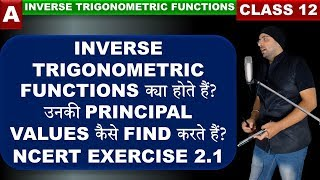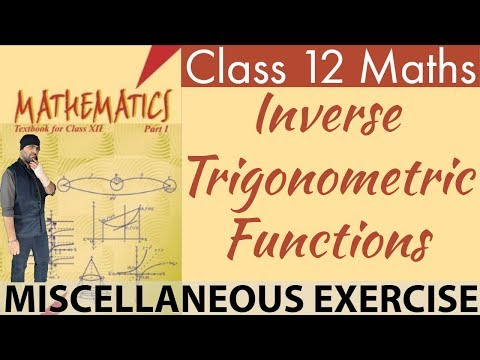We provide expert created educational resources to resolute the inflexible educational standards and provide high quality animated free video lectures, pdf notes and assignments for Inverse Trigonometric Functions Class 12 Maths while offering you the convenience and flexibility of free online tuition.

We have developed comprehensive and detailed study materials for Class 12 Maths. It covers all the basic concepts, including the advanced concepts from the boards point of view.

Summary:

The ranges (principal value branches) of inverse trigonometric functions are given in the following table:

 Functions Range $$\sin^{-1}x$$ $$\left[ \frac{-\pi}{2}, \frac{\pi}{2} \right]$$ $$\csc^{-1}x$$ $$\left[ \frac{-\pi}{2}, \frac{\pi}{2} \right] – {0}$$ $$\tan^{-1}x$$ $$\left( \frac{-\pi}{2}, \frac{\pi}{2} \right)$$ $$\cos^{-1}x$$ $$[0, \pi]$$ $$\sec^{-1}x$$ $$[0, \pi] – { \frac{\pi}{2} }$$ $$\cot^{-1}x$$ $$(0, \pi)$$

$$\sin^{-1}x$$ should not be confused with $$(\sin x)^{-1}$$. In fact $$(\sin x)^{-1} = \frac{1}{\sin x}$$ and similarly for other trigonometric functions.

The value of an inverse trigonometric functions which lies in its principal value branch is called the principal value of that inverse trigonometric functions.